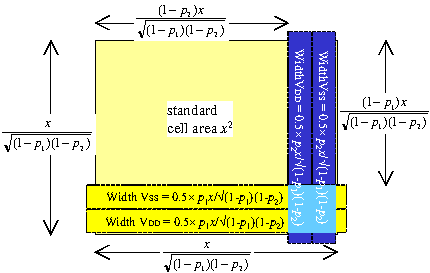This consideration applies to standard cells (like the vsclib) which use metal-1 running horizontally as the power supplies. We define ps as the fraction of metal‑1 used for the power supplies, and this value is measured from the standard cell itself (see the standard cell page). A typical value is 25%.

First we consider the case of a square standard cell core with no power straps, and then extend the argument to a square core with power straps.

The voltage applied to the chip core is Vpad and the core power consumption is Vpad×Iext. This is related to the power at nominal supply voltage by

We have seen that when the entire metal layer is used for power supplies, Iext = (VpadVmin) × G(n). In the case here where we use the fraction ps of metal-1 for power supply routing, Iext = (VpadVmin) × ps × G(1).

The power is only delivered through the standard cells' power rails, which means that Ptot = P(S). Replacing Iext in the expression for Ptot above gives

Referring to the drawing on the right, to the standard cell area x×x we add horizontal power straps in metal-1 and vertical power straps in metal-2. We keep the core square.

Web page under construction.

Design Attributes
Ptot core power consumption defined at nominal supply voltage
ps the fraction of metal-1 in the standard cells used for the power supplies
r(1) metal-1 resistivity measured in ohms per square
G(1) power supply conductance for metal-1 ( =7/(4×r(1)) )
Vdd the nominal supply voltage
Vpad the minimum voltage at the edge of the core
Vmin the minimum desired voltage at the centre of the die
Iext the core current when the voltage at the edge of the core is Vpad